# Class quaternion Documentation

Quaternions are a relative of complex numbers.

Quaternions are in fact part of a small hierarchy of structures built upon the real numbers, which comprise only the set of real numbers (traditionally named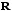), the set of complex numbers (traditionally named), the set of quaternions (traditionally named) and the set of octonions (traditionally named), which possess interesting mathematical properties (chief among which is the fact that they are division algebras, i.e. where the following property is true: ifis an element of that algebra and is not equal to zero, then, where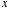anddenote elements of that algebra, implies that). Each member of the hierarchy is a super-set of the former.

One of the most important aspects of quaternions is that they provide an efficient way to parameterize rotations in(the usual three-dimensional space) and.

In practical terms, a quaternion is simply a quadruple of real numbers, which we can write in the form, whereis the same object as for complex numbers, and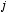andare distinct objects which play essentially the same kind of role as.

An addition and a multiplication is defined on the set of quaternions, which generalize their real and complex counterparts. The main novelty here is that the multiplication is not commutative (i.e. there are quaternionsandsuch that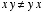). A good mnemotechnical way of remembering things is by using the formula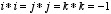.

Quaternions (and their kin) are described in far more details in this other document (with errata and addenda).

Some traditional constructs, such as the exponential, carry over without too much change into the realms of quaternions, but other, such as taking a square root, do not.

## Acknowledgements

The mathematical text has been typeset with Nisus Writer. Jens Maurer has helped with portability and standard adherence, and was the Review Manager for this library. More acknowledgements in the History section. Thank you to all who contributed to the discution about this library.

The interface and implementation are both supplied by the header file quaternion.h.

## Test Program

The `quaternion_test.cpp` test program tests quaternions specializations for float, double and long double (sample output, with message output enabled).

If you define the symbol `BOOST_QUATERNION_TEST_VERBOSE`, you will get additional output (verbose output); this will only be helpfull if you enable message output at the same time, of course (by uncommenting the relevant line in the test or by adding `--log_level=messages` to your command line,...). In that case, and if you are running interactively, you may in addition define the symbol `BOOST_INTERACTIVE_TEST_INPUT_ITERATOR` to interactively test the input operator with input of your choice from the standard input (instead of hard-coding it in the test).

## Synopsis

``````namespace boost
{

namespace math
{

template<typename T> class quaternion;
template<>           class quaternion<float>;
template<>           class quaternion<double>;
template<>           class quaternion<long double>;

// operators

template<typename T> inline quaternion<T> operator + (T const & lhs, quaternion<T> const & rhs);
template<typename T> inline quaternion<T> operator + (quaternion<T> const & lhs, T const & rhs);
template<typename T> inline quaternion<T> operator + (::std::complex<T> const & lhs, quaternion<T> const & rhs);
template<typename T> inline quaternion<T> operator + (quaternion<T> const & lhs, ::std::complex<T> const & rhs);
template<typename T> inline quaternion<T> operator + (quaternion<T> const & lhs, quaternion<T> const & rhs);

template<typename T> inline quaternion<T> operator - (T const & lhs, quaternion<T> const & rhs);
template<typename T> inline quaternion<T> operator - (quaternion<T> const & lhs, T const & rhs);
template<typename T> inline quaternion<T> operator - (::std::complex<T> const & lhs, quaternion<T> const & rhs);
template<typename T> inline quaternion<T> operator - (quaternion<T> const & lhs, ::std::complex<T> const & rhs);
template<typename T> inline quaternion<T> operator - (quaternion<T> const & lhs, quaternion<T> const & rhs);

template<typename T> inline quaternion<T> operator * (T const & lhs, quaternion<T> const & rhs);
template<typename T> inline quaternion<T> operator * (quaternion<T> const & lhs, T const & rhs);
template<typename T> inline quaternion<T> operator * (::std::complex<T> const & lhs, quaternion<T> const & rhs);
template<typename T> inline quaternion<T> operator * (quaternion<T> const & lhs, ::std::complex<T> const & rhs);
template<typename T> inline quaternion<T> operator * (quaternion<T> const & lhs, quaternion<T> const & rhs);

template<typename T> inline quaternion<T> operator / (T const & lhs, quaternion<T> const & rhs);
template<typename T> inline quaternion<T> operator / (quaternion<T> const & lhs, T const & rhs);
template<typename T> inline quaternion<T> operator / (::std::complex<T> const & lhs, quaternion<T> const & rhs);
template<typename T> inline quaternion<T> operator / (quaternion<T> const & lhs, ::std::complex<T> const & rhs);
template<typename T> inline quaternion<T> operator / (quaternion<T> const & lhs, quaternion<T> const & rhs);

template<typename T> inline quaternion<T> operator + (quaternion<T> const & q);
template<typename T> inline quaternion<T> operator - (quaternion<T> const & q);

template<typename T> inline bool operator == (T const & lhs, quaternion<T> const & rhs);
template<typename T> inline bool operator == (quaternion<T> const & lhs, T const & rhs);
template<typename T> inline bool operator == (::std::complex<T> const & lhs, quaternion<T> const & rhs);
template<typename T> inline bool operator == (quaternion<T> const & lhs, ::std::complex<T> const & rhs);
template<typename T> inline bool operator == (quaternion<T> const & lhs, quaternion<T> const & rhs);

template<typename T> inline bool operator != (T const & lhs, quaternion<T> const & rhs);
template<typename T> inline bool operator != (quaternion<T> const & lhs, T const & rhs);
template<typename T> inline bool operator != (::std::complex<T> const & lhs, quaternion<T> const & rhs);
template<typename T> inline bool operator != (quaternion<T> const & lhs, ::std::complex<T> const & rhs);
template<typename T> inline bool operator != (quaternion<T> const & lhs, quaternion<T> const & rhs);

template<typename T, typename charT, class traits>
::std::basic_istream<charT,traits> &    operator >> (::std::basic_istream<charT,traits> & is, quaternion<T> & q);

template<typename T, typename charT, class traits>
::std::basic_ostream<charT,traits> &    operator << (::std::basic_ostream<charT,traits> & os, quaternion<T> const & q);

// values

template<typename T>    inline T        real(quaternion<T> const & q);
template<typename T>    inline quaternion<T>    unreal(quaternion<T> const & q);

template<typename T>    inline T        sup(quaternion<T> const & q);
template<typename T>    inline T        l1(quaternion<T> const & q);
template<typename T>    inline T        abs(quaternion<T> const & q);
template<typename T>    inline T        norm(quaternion<T>const  & q);
template<typename T>    inline quaternion<T>    conj(quaternion<T> const & q);

template<typename T>    inline quaternion<T>    spherical(T const & rho, T const & theta, T const & phi1, T const & phi2);
template<typename T>    inline quaternion<T>    semipolar(T const & rho, T const & alpha, T const & theta1, T const & theta2);
template<typename T>    inline quaternion<T>    multipolar(T const & rho1, T const & theta1, T const & rho2, T const & theta2);
template<typename T>    inline quaternion<T>    cylindrospherical(T const & t, T const & radius, T const & longitude, T const & latitude);
template<typename T>    inline quaternion<T>    cylindrical(T const & r, T const & angle, T const & h1, T const & h2);

// transcendentals

template<typename T>    inline quaternion<T>    exp(quaternion<T> const & q);
template<typename T>    inline quaternion<T>    cos(quaternion<T> const & q);
template<typename T>    inline quaternion<T>    sin(quaternion<T> const & q);
template<typename T>    inline quaternion<T>    tan(quaternion<T> const & q);
template<typename T>    inline quaternion<T>    cosh(quaternion<T> const & q);
template<typename T>    inline quaternion<T>    sinh(quaternion<T> const & q);
template<typename T>    inline quaternion<T>    tanh(quaternion<T> const & q);

template<typename T>    quaternion<T>       pow(quaternion<T> const & q, int n);

}

}
``````

## Template class `quaternion`

``````namespace boost
{
namespace math
{

template<typename T>
class quaternion
{
public:

typedef T value_type;

explicit        quaternion(T const & requested_a = T(), T const & requested_b = T(), T const & requested_c = T(), T const & requested_d = T());
explicit        quaternion(::std::complex<T> const & z0, ::std::complex<T> const & z1 = ::std::complex<T>());
template<typename X> explicit   quaternion(quaternion<X> const & a_recopier);

T           real() const;
quaternion<T>       unreal() const;
T           R_component_1() const;
T           R_component_2() const;
T           R_component_3() const;
T           R_component_4() const;
::std::complex<T>   C_component_1() const;
::std::complex<T>   C_component_2() const;

quaternion<T> & operator = (quaternion<T> const  & a_affecter);
template<typename X>    quaternion<T> & operator = (quaternion<X> const  & a_affecter);
quaternion<T> & operator = (T const  & a_affecter);
quaternion<T> & operator = (::std::complex<T> const & a_affecter);

quaternion<T> & operator += (T const & rhs);
quaternion<T> & operator += (::std::complex<T> const & rhs);
template<typename X>    quaternion<T> & operator += (quaternion<X> const & rhs);

quaternion<T> & operator -= (T const & rhs);
quaternion<T> & operator -= (::std::complex<T> const & rhs);
template<typename X>    quaternion<T> & operator -= (quaternion<X> const & rhs);

quaternion<T> & operator *= (T const & rhs);
quaternion<T> & operator *= (::std::complex<T> const & rhs);
template<typename X>    quaternion<T> & operator *= (quaternion<X> const & rhs);

quaternion<T> & operator /= (T const & rhs);
quaternion<T> & operator /= (::std::complex<T> const & rhs);
template<typename X>    quaternion<T> & operator /= (quaternion<X> const & rhs);

};

}

}
``````

## `quaternion` specializations

``````namespace boost
{

namespace math
{

template<>
class quaternion<float>
{
public:

typedef float value_type;

explicit        quaternion(float const & requested_a = 0.0f, float const & requested_b = 0.0f, float const & requested_c = 0.0f, float const & requested_d = 0.0f);
explicit        quaternion(::std::complex<float> const & z0, ::std::complex<float> const & z1 = ::std::complex<float>());
explicit        quaternion(quaternion<double> const & a_recopier);
explicit        quaternion(quaternion<long double> const & a_recopier);

float           real() const;
quaternion<float>   unreal() const;
float           R_component_1() const;
float           R_component_2() const;
float           R_component_3() const;
float           R_component_4() const;
::std::complex<float>   C_component_1() const;
::std::complex<float>   C_component_2() const;

quaternion<float> & operator = (quaternion<float> const & a_affecter);
template<typename X>    quaternion<float> & operator = (quaternion<X>const  & a_affecter);
quaternion<float> & operator = (float const & a_affecter);
quaternion<float> & operator = (::std::complex<float> const & a_affecter);

quaternion<float> & operator += (float const & rhs);
quaternion<float> & operator += (::std::complex<float> const & rhs);
template<typename X>    quaternion<float> & operator += (quaternion<X> const & rhs);

quaternion<float> & operator -= (float const & rhs);
quaternion<float> & operator -= (::std::complex<float> const & rhs);
template<typename X>    quaternion<float> & operator -= (quaternion<X> const & rhs);

quaternion<float> & operator *= (float const & rhs);
quaternion<float> & operator *= (::std::complex<float> const & rhs);
template<typename X>    quaternion<float> & operator *= (quaternion<X> const & rhs);

quaternion<float> & operator /= (float const & rhs);
quaternion<float> & operator /= (::std::complex<float> const & rhs);
template<typename X>    quaternion<float> & operator /= (quaternion<X> const & rhs);

};

template<>
class quaternion<double>
{
public:

typedef double value_type;

explicit        quaternion(double const & requested_a = 0.0, double const & requested_b = 0.0, double const & requested_c = 0.0, double const & requested_d = 0.0);
explicit        quaternion(::std::complex<double> const & z0, ::std::complex<double> const & z1 = ::std::complex<double>());
explicit        quaternion(quaternion<float> const & a_recopier);
explicit        quaternion(quaternion<long double> const & a_recopier);

double          real() const;
quaternion<double>  unreal() const;
double          R_component_1() const;
double          R_component_2() const;
double          R_component_3() const;
double          R_component_4() const;
::std::complex<double>  C_component_1() const;
::std::complex<double>  C_component_2() const;

quaternion<double> &    operator = (quaternion<double> const & a_affecter);
template<typename X>    quaternion<double> &    operator = (quaternion<X> const & a_affecter);
quaternion<double> &    operator = (double const & a_affecter);
quaternion<double> &    operator = (::std::complex<double> const & a_affecter);

quaternion<double> &    operator += (double const & rhs);
quaternion<double> &    operator += (::std::complex<double> const & rhs);
template<typename X> quaternion<double> &   operator += (quaternion<X> const & rhs);

quaternion<double> &    operator -= (double const & rhs);
quaternion<double> &    operator -= (::std::complex<double> const & rhs);
template<typename X> quaternion<double> &   operator -= (quaternion<X> const & rhs);

quaternion<double> &    operator *= (double const & rhs);
quaternion<double> &    operator *= (::std::complex<double> const & rhs);
template<typename X> quaternion<double> &   operator *= (quaternion<X> const & rhs);

quaternion<double> &    operator /= (double const & rhs);
quaternion<double> &    operator /= (::std::complex<double> const & rhs);
template<typename X>    quaternion<double> &    operator /= (quaternion<X> const & rhs);

};

template<>
class quaternion<long double>
{
public:

typedef long double value_type;

explicit            quaternion(long double const & requested_a = 0.0L, long double const & requested_b = 0.0L, long double const & requested_c = 0.0L, long double const & requested_d = 0.0L);
explicit            quaternion( ::std::complex<long double> const & z0, ::std::complex<long double> const & z1 = ::std::complex<long double>());
explicit            quaternion(quaternion<float> const & a_recopier);
explicit            quaternion(quaternion<double> const & a_recopier);

long double         real() const;
quaternion<long double>     unreal() const;
long double         R_component_1() const;
long double         R_component_2() const;
long double         R_component_3() const;
long double         R_component_4() const;
::std::complex<long double> C_component_1() const;
::std::complex<long double> C_component_2() const;

quaternion<long double> &   operator = (quaternion<long double> const & a_affecter);
template<typename X>    quaternion<long double> &   operator = (quaternion<X> const & a_affecter);
quaternion<long double> &   operator = (long double const & a_affecter);
quaternion<long double> &   operator = (::std::complex<long double> const & a_affecter);

quaternion<long double> &   operator += (long double const & rhs);
quaternion<long double> &   operator += (::std::complex<long double> const & rhs);
template<typename X>    quaternion<long double> &   operator += (quaternion<X> const & rhs);

quaternion<long double> &   operator -= (long double const & rhs);
quaternion<long double> &   operator -= (::std::complex<long double> const & rhs);
template<typename X>    quaternion<long double> &   operator -= (quaternion<X> const & rhs);

quaternion<long double> &   operator *= (long double const & rhs);
quaternion<long double> &   operator *= (::std::complex<long double> const & rhs);
template<typename X>    quaternion<long double> &   operator *= (quaternion<X> const & rhs);

quaternion<long double> &   operator /= (long double const & rhs);
quaternion<long double> &   operator /= (::std::complex<long double> const & rhs);
template<typename X>    quaternion<long double> &   operator /= (quaternion<X> const & rhs);

};

}

}
``````

## `quaternion` typedefs

### `value_type`

• `typedef T value_type;`
• Template version.
• `typedef float value_type;`
• Float specialization version.
• `typedef double value_type;`
• Double specialization version.
• `typedef long double value_type;`
• Long double specialization version.
• These provide easy acces to the type the template is built upon.

## `quaternion` member functions

### Constructors

``````explicit    quaternion(T const & requested_a = T(), T const & requested_b = T(), T const & requested_c = T(), T const & requested_d = T());
explicit    quaternion(::std::complex<T> const & z0, ::std::complex<T> const & z1 = ::std::complex<T>());
template<typename X>
explicit    quaternion(quaternion<X> const & a_recopier);
``````

Template version.

``````explicit    quaternion(float const & requested_a = 0.0f, float const & requested_b = 0.0f, float const & requested_c = 0.0f, float const & requested_d = 0.0f);
explicit    quaternion(::std::complex<float> const & z0,::std::complex<float> const & z1 = ::std::complex<float>());
explicit    quaternion(quaternion<double> const & a_recopier);
explicit    quaternion(quaternion<long double> const & a_recopier);
``````

Float specialization version.

``````explicit    quaternion(double const & requested_a = 0.0, double const & requested_b = 0.0, double const & requested_c = 0.0, double const & requested_d = 0.0);
explicit    quaternion(::std::complex<double> const & z0, ::std::complex<double> const & z1 = ::std::complex<double>());
explicit    quaternion(quaternion<float> const & a_recopier);
explicit    quaternion(quaternion<long double> const & a_recopier);
``````

Double specialization version.

``````explicit    quaternion(long double const & requested_a = 0.0L, long double const & requested_b = 0.0L, long double const & requested_c = 0.0L, long double const & requested_d = 0.0L);
explicit    quaternion( ::std::complex<long double> const & z0, ::std::complex<long double> const & z1 = ::std::complex<long double>());
explicit    quaternion(quaternion<float> const & a_recopier);
explicit    quaternion(quaternion<double> const & a_recopier);
``````

Long double specialization version.

A default constructor is provided for each form, which initializes each component to the default values for their type (i.e. zero for floating numbers). This constructor can also accept one to four base type arguments. A constructor is also provided to build quaternions from one or two complex numbers sharing the same base type. The unspecialized template also sports a templarized copy constructor, while the specialized forms have copy constructors from the other two specializations, which are explicit when a risk of precision loss exists. For the unspecialized form, the base type's constructors must not throw.

Destructors and untemplated copy constructors (from the same type) are provided by the compiler. Converting copy constructors make use of a templated helper function in a "detail" subnamespace.

### Other member functions

``````T       real() const;
quaternion<T>   unreal() const;
``````

Like complex number, quaternions do have a meaningful notion of "real part", but unlike them there is no meaningful notion of "imaginary part". Instead there is an "unreal part" which itself is a quaternion, and usually nothing simpler (as opposed to the complex number case). These are returned by the first two functions.

``````T   R_component_1() const;
T   R_component_2() const;
T   R_component_3() const;
T   R_component_4() const;
``````

A quaternion having four real components, these are returned by these four functions. Hence `real` and `R_component_1` return the same value.

``````::std::complex<T>   C_component_1() const;
::std::complex<T>   C_component_2() const;
``````

A quaternion likewise has two complex components, and as we have seen above, for any quaternion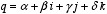we also have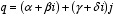These functions return them. The real part of `q.C_component_1()` is the same as `q.real()`.

## `quaternion` member operators

### Assignment operators

``````quaternion<T> & operator = (quaternion<T> const & a_affecter);
template<typename X> quaternion<T> & operator = (quaternion<X> const & a_affecter);
quaternion<T> & operator = (T const & a_affecter);
quaternion<T> & operator = (::std::complex<T> const & a_affecter);
``````

These perform the expected assignment, with type modification if necessary (for instance, assigning from a base type will set the real part to that value, and all other components to zero). For the unspecialized form, the base type's assignment operators must not throw.

### Other member operators

``````quaternion<T> & operator += (T const & rhs)
quaternion<T> & operator += (::std::complex<T> const & rhs);
template<typename X>    quaternion<T> & operator += (quaternion<X> const & rhs);
``````

These perform the mathematical operation `(*this)+rhs` and store the result in `*this`. The unspecialized form has exception guards, which the specialized forms do not, so as to insure exception safety. For the unspecialized form, the base type's assignment operators must not throw.

``````quaternion<T> & operator -= (T const & rhs)
quaternion<T> & operator -= (::std::complex<T> const & rhs);
template<typename X>    quaternion<T> & operator -= (quaternion<X> const & rhs);
``````

These perform the mathematical operation `(*this)-rhs` and store the result in `*this`. The unspecialized form has exception guards, which the specialized forms do not, so as to insure exception safety. For the unspecialized form, the base type's assignment operators must not throw.

``````quaternion<T> & operator *= (T const & rhs)
quaternion<T> & operator *= (::std::complex<T> const & rhs);
template<typename X>    quaternion<T> & operator *= (quaternion<X> const & rhs);
``````

These perform the mathematical operation `(*this)*rhs` in this order (order is important as multiplication is not commutative for quaternions) and store the result in `*this`. The unspecialized form has exception guards, which the specialized forms do not, so as to insure exception safety. For the unspecialized form, the base type's assignment operators must not throw.

``````quaternion<T> & operator /= (::std::complex<T> const & rhs);
template<typename X>    quaternion<T> & operator /= (quaternion<X> const & rhs);
``````

These perform the mathematical operation `(*this)*inverse_of(rhs)` in this order (order is important as multiplication is not commutative for quaternions) and store the result in `*this`. The unspecialized form has exception guards, which the specialized forms do not, so as to insure exception safety. For the unspecialized form, the base type's assignment operators must not throw.

## `quaternion` non-member operations

``````template<typename T>    inline quaternion<T>    operator + (quaternion<T> const & q);
``````

This unary operator simply returns `q`.

``````template<typename T>    inline quaternion<T>    operator - (quaternion<T> const & q);
``````

This unary operator returns the opposite of `q`.

``````template<typename T>    inline quaternion<T>    operator + (T const & lhs, quaternion<T> const & rhs);
template<typename T>    inline quaternion<T>    operator + (quaternion<T> const & lhs, T const & rhs);
template<typename T>    inline quaternion<T>    operator + (::std::complex<T> const & lhs, quaternion<T> const & rhs);
template<typename T>    inline quaternion<T>    operator + (quaternion<T> const & lhs, ::std::complex<T> const & rhs);
template<typename T>    inline quaternion<T>    operator + (quaternion<T> const & lhs, quaternion<T> const & rhs);
``````

These operators return `quaternion<T>(lhs) += rhs`.

``````template<typename T>    inline quaternion<T>    operator - (quaternion<T> const & lhs, T const & rhs);
template<typename T>    inline quaternion<T>    operator - (::std::complex<T> const & lhs, quaternion<T> const & rhs);
template<typename T>    inline quaternion<T>    operator - (quaternion<T> const & lhs, ::std::complex<T> const & rhs);
template<typename T>    inline quaternion<T>    operator - (quaternion<T> const & lhs, quaternion<T> const & rhs);
``````

These operators return `quaternion<T>(lhs) -= rhs`.

``````template<typename T>    inline quaternion<T>    operator * (T const & lhs, quaternion<T> const & rhs);
template<typename T>    inline quaternion<T>    operator * (quaternion<T> const & lhs, T const & rhs);
template<typename T>    inline quaternion<T>    operator * (::std::complex<T> const & lhs, quaternion<T> const & rhs);
template<typename T>    inline quaternion<T>    operator * (quaternion<T> const & lhs, ::std::complex<T> const & rhs);
template<typename T>    inline quaternion<T>    operator * (quaternion<T> const & lhs, quaternion<T> const & rhs);
``````

These operators return `quaternion<T>(lhs) *= rhs`.

``````template<typename T>    inline quaternion<T>    operator / (T const & lhs, quaternion<T> const & rhs);
template<typename T>    inline quaternion<T>    operator / (quaternion<T> const & lhs, T const & rhs);
template<typename T>    inline quaternion<T>    operator / (::std::complex<T> const & lhs, quaternion<T> const & rhs);
template<typename T>    inline quaternion<T>    operator / (quaternion<T> const & lhs, ::std::complex<T> const & rhs);
template<typename T>    inline quaternion<T>    operator / (quaternion<T> const & lhs, quaternion<T> const & rhs);
``````

These operators return `quaternion<T>(lhs) /= rhs`. It is of course still an error to divide by zero...

``````template<typename T>    inline bool operator == (T const & lhs, quaternion<T> const & rhs);
template<typename T>    inline bool operator == (quaternion<T> const & lhs, T const & rhs);
template<typename T>    inline bool operator == (::std::complex<T> const & lhs, quaternion<T> const & rhs);
template<typename T>    inline bool operator == (quaternion<T> const & lhs, ::std::complex<T> const & rhs);
template<typename T>    inline bool operator == (quaternion<T> const & lhs, quaternion<T> const & rhs);
``````

These return `true` if and only if the four components of `quaternion<T>(lhs)` are equal to their counterparts in `quaternion<T>(rhs)`. As with any floating-type entity, this is essentially meaningless.

``````template<typename T>    inline bool operator != (T const & lhs, quaternion<T> const & rhs);
template<typename T>    inline bool operator != (quaternion<T> const & lhs, T const & rhs);
template<typename T>    inline bool operator != (::std::complex<T> const & lhs, quaternion<T> const & rhs);
template<typename T>    inline bool operator != (quaternion<T> const & lhs, ::std::complex<T> const & rhs);
template<typename T>    inline bool operator != (quaternion<T> const & lhs, quaternion<T> const & rhs);
``````

These return `true` if and only if `quaternion<T>(lhs) == quaternion<T>(rhs)` is `false`. As with any floating-type entity, this is essentially meaningless.

``````template<typename T, typename charT, class traits>
::std::basic_istream<charT,traits> &    operator >> (::std::basic_istream<charT,traits> & is, quaternion<T> & q);
``````

Extracts a quaternion `q` of one of the following forms (with a, b, c and d of type `T`):

`a (a), (a,b), (a,b,c), (a,b,c,d) (a,(c)), (a,(c,d)), ((a)), ((a),c), ((a),(c)), ((a),(c,d)), ((a,b)), ((a,b),c), ((a,b),(c)), ((a,b),(c,d))`

The input values must be convertible to T. If bad input is encountered, calls `is.setstate(ios::failbit)` (which may throw ios::failure (27.4.5.3)).

Returns `is`.

The rationale for the list of accepted formats is that either we have a list of up to four reals, or else we have a couple of complex numbers, and in that case if it formated as a proper complex number, then it should be accepted. Thus potential ambiguities are lifted (for instance (a,b) is (a,b,0,0) and not (a,0,b,0), i.e. it is parsed as a list of two real numbers and not two complex numbers which happen to have imaginary parts equal to zero).

``````template<typename T, typename charT, class traits>
::std::basic_ostream<charT,traits> &    operator << (::std::basic_ostream<charT,traits> & os, quaternion<T> const & q);
``````

Inserts the quaternion `q` onto the stream `os` as if it were implemented as follows:

``````template<typename T, typename charT, class traits>
::std::basic_ostream<charT,traits> &    operator << (   ::std::basic_ostream<charT,traits> & os,
quaternion<T> const & q)
{
::std::basic_ostringstream<charT,traits>    s;

s.flags(os.flags());
s.imbue(os.getloc());
s.precision(os.precision());

s << '('    << q.R_component_1() << ','
<< q.R_component_2() << ','
<< q.R_component_3() << ','
<< q.R_component_4() << ')';

return os << s.str();
}
``````

## `quaternion` value operations

``````template<typename T>    inline T        real(quaternion<T> const & q);
template<typename T>    inline quaternion<T>    unreal(quaternion<T> const & q);
``````

These return `q.real()` and `q.unreal()` respectively.

``````template<typename T>    inline quaternion<T>    conj(quaternion<T> const & q);
``````

This returns the conjugate of the quaternion.

``````template<typename T>    inline T        sup(quaternion<T> const & q);
``````

This return the sup norm (the greatest among `abs(q.R_component_1())`...`abs(q.R_component_4())`) of the quaternion.

``````template<typename T>    inline T        l1(quaternion<T> const & q);
``````

This return the l1 norm (`abs(q.R_component_1())+...+abs(q.R_component_4())`) of the quaternion.

``````template<typename T>    inline T        abs(quaternion<T> const & q);
``````

This return the magnitude (Euclidian norm) of the quaternion.

``````template<typename T>    inline T        norm(quaternion<T>const  & q);
``````

This return the (Cayley) norm of the quaternion. The term "norm" might be confusing, as most people associate it with the Euclidian norm (and quadratic functionals). For this version of (the mathematical objects known as) quaternions, the Euclidian norm (also known as magnitude) is the square root of the Cayley norm.

``````template<typename T>    inline quaternion<T>    spherical(T const & rho, T const & theta, T const & phi1, T const & phi2);
template<typename T>    inline quaternion<T>    semipolar(T const & rho, T const & alpha, T const & theta1, T const & theta2);
template<typename T>    inline quaternion<T>    multipolar(T const & rho1, T const & theta1, T const & rho2, T const & theta2);
template<typename T>    inline quaternion<T>    cylindrospherical(T const & t, T const & radius, T const & longitude, T const & latitude);
template<typename T>    inline quaternion<T>    cylindrical(T const & r, T const & angle, T const & h1, T const & h2);
``````

These build quaternions in a way similar to the way `polar` builds complex numbers, as there is no strict equivalent to polar coordinates for quaternions.

`spherical` is a simple transposition of `polar`, it takes as inputs a (positive) magnitude and a point on the hypersphere, given by three angles. The first of these, `theta` has a natural range of `-pi` to `+pi`, and the other two have natural ranges of `-pi/2` to `+pi/2` (as is the case with the usual spherical coordinates in R^3). Due to the many symmetries and periodicities, nothing untoward happens if the magnitude is negative or the angles are outside their natural ranges. The expected degeneracies (a magnitude of zero ignores the angles settings...) do happen however.

`cylindrical` is likewise a simple transposition of the usual cylindrical coordinates in R^3, which in turn is another derivative of planar polar coordinates. The first two inputs are the polar coordinates of the first C component of the quaternion. The third and fourth inputs are placed into the third and fourth R components of the quaternion, respectively.

`multipolar` is yet another simple generalization of polar coordinates. This time, both C components of the quaternion are given in polar coordinates.

`cylindrospherical` is specific to quaternions. It is often interesting to consider H as the cartesian product of R by R^3 (the quaternionic multiplication as then a special form, as given here). This function therefore builds a quaternion from this representation, with the R^3 component given in usual R^3 spherical coordinates.

`semipolar` is another generator which is specific to quaternions. It takes as a first input the magnitude of the quaternion, as a second input an angle in the range `0` to `+pi/2` such that magnitudes of the first two C components of the quaternion are the product of the first input and the sine and cosine of this angle, respectively, and finally as third and fourth inputs angles in the range `-pi/2` to `+pi/2` which represent the arguments of the first and second C components of the quaternion, respectively. As usual, nothing untoward happens if what should be magnitudes are negative numbers or angles are out of their natural ranges, as symmetries and periodicities kick in.

In this version of our implementation of quaternions, there is no analogue of the complex value operation `arg` as the situation is somewhat more complicated. Unit quaternions are linked both to rotations in R^3 and in R^4, and the correspondences are not too complicated, but there is currently a lack of standard (de facto or de jure) matrix library with which the conversions could work. This should be remedied in a further revision. In the mean time, an example of how this could be done is presented here for R^3, and here for R^4 (example test file).

## `quaternion` transcendentals

There is no `log` or `sqrt` provided for quaternions in this implementation, and `pow` is likewise restricted to integral powers of the exponent. There are several reasons to this: on the one hand, the equivalent of analytic continuation for quaternions ("branch cuts") remains to be investigated thoroughly (by me, at any rate...), and we wish to avoid the nonsense introduced in the standard by exponentiations of complexes by complexes (which is well defined, but not in the standard...). Talking of nonsense, saying that `pow(0,0)` is "implementation defined" is just plain brain-dead...

We do, however provide several transcendentals, chief among which is the exponential. This author claims the complete proof of the "closed formula" as his own, as well as its independant invention (there are claims to prior invention of the formula, such as one by Professor Shoemake, and it is possible that the formula had been known a couple of centuries back, but in absence of bibliographical reference, the matter is pending, awaiting further investigation; on the other hand, the definition and existence of the exponential on the quaternions, is of course a fact known for a very long time). Basically, any converging power series with real coefficients which allows for a closed formula in C can be transposed to H. More transcendentals of this type could be added in a further revision upon request. It should be noted that it is these functions which force the dependency upon the `boost/math/special_functions/sinc.hpp` and the `boost/math/special_functions/sinhc.hpp` headers.

### exp

``````template<typename T>    inline quaternion<T>    exp(quaternion<T> const & q);
``````

Computes the exponential of the quaternion.

### cos

``````template<typename T>    inline quaternion<T>    cos(quaternion<T> const & q);
``````

Computes the cosine of the quaternion

### sin

``````template<typename T>    inline quaternion<T>    sin(quaternion<T> const & q);
``````

Computes the sine of the quaternion.

### tan

``````template<typename T>    inline quaternion<T>    tan(quaternion<T> const & q);
``````

Computes the tangent of the quaternion.

### cosh

``````template<typename T>    inline quaternion<T>    cosh(quaternion<T> const & q);
``````

Computes the hyperbolic cosine of the quaternion.

### sinh

``````template<typename T>    inline quaternion<T>    sinh(quaternion<T> const & q);
``````

Computes the hyperbolic sine of the quaternion.

### tanh

``````template<typename T>    inline quaternion<T>    tanh(quaternion<T> const & q);
``````

Computes the hyperbolic tangent of the quaternion.

### pow

``````_emplate<typename T>    quaternion<T>       pow(quaternion<T> const & q, int n);
``````

Computes the `n`-th power of the quaternion `q`.

## History

• 1.5.7 - 24/02/2003: transitionned to the unit test framework; `<boost/config.hpp>` now included by the library header (rather than the test files).
• 1.5.6 - 15/10/2002: Gcc2.95.x and stlport on linux compatibility by Alkis Evlogimenos (alkis@routescience.com).
• 1.5.5 - 27/09/2002: Microsoft VCPP 7 compatibility, by Michael Stevens (michael@acfr.usyd.edu.au); requires the /Za compiler option.
• 1.5.4 - 19/09/2002: fixed problem with multiple inclusion (in different translation units); attempt at an improved compatibility with Microsoft compilers, by Michael Stevens (michael@acfr.usyd.edu.au) and Fredrick Blomqvist (fredrik@blomqvist.nu); other compatibility fixes.
• 1.5.3 - 01/02/2002: bugfix and Gcc 2.95.3 compatibility by Douglas Gregor (gregod@cs.rpi.edu).
• 1.5.2 - 07/07/2001: introduced `namespace math`.
• 1.5.1 - 07/06/2001: (end of Boost review) now includes `<boost/math/special_functions/sinc.hpp>` and `<boost/math/special_functions/sinhc.hpp>` instead of `<boost/special_functions.hpp>`; corrected bug in `sin` (Daryle Walker); removed check for self-assignment (Gary Powel); made converting functions `explicit` (Gary Powel); added overflow guards for division operators and `abs` (Peter Schmitteckert); added `sup` and `l1`; used Vesa Karvonen's CPP metaprograming technique to simplify code.
• 1.5.0 - 26/03/2001: boostification, inlining of all operators except input, output and `pow`, fixed exception safety of some members (template version) and output operator, added `spherical`, `semipolar`, `multipolar`, `cylindrospherical` and `cylindrical`.
• 1.4.0 - 09/01/2001: added `tan` and `tanh`.
• 1.3.1 - 08/01/2001: cosmetic fixes.
• 1.3.0 - 12/07/2000: `pow` now uses Maarten Hilferink's (mhilferink@tip.nl) algorithm.
• 1.2.0 - 25/05/2000: fixed the division operators and output; changed many signatures.
• 1.1.0 - 23/05/2000: changed `sinc` into `sinc_pi`; added `sin`, `cos`, `sinh`, `cosh`.
• 1.0.0 - 10/08/1999: first public version.

## To Do

• Improve testing.
• Rewrite input operatore using Spirit (creates a dependency).
• Put in place an Expression Template mechanism (perhaps borrowing from uBlas).
• Use uBlas for the link with rotations (and move from the example implementation to an efficient one).

Revised 24 Feb 2003 (c) Copyright Hubert Holin 2001-2003. Permission to copy, use, modify, sell and distribute this document is granted provided this copyright notice appears in all copies. This software is provided "as is" without express or implied warranty, and with no claim as to its suitability for any purpose.